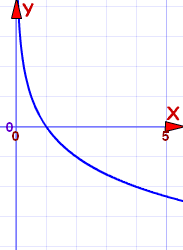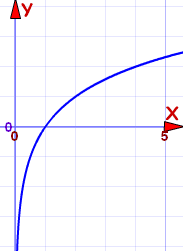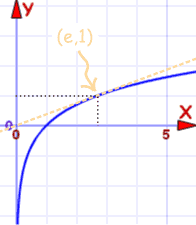# Logarithmic Function Reference

This is the Logarithmic Function:

f(x) = loga(x)

a is any value greater than 0, except 1

### Properties depend on value of "a"

• When a=1, the graph is not defined
• Apart from that there are two cases to look at:

### a above 1Example: f(x) = log½(x)

Example: f(x) = log2(x)

For a between 0 and 1

For a above 1:

Plot the graph here (use the "a" slider)

### In general, the logarithmic function:

• always has positive x, and never crosses the y-axis
• always intersects the x-axis at x=1 ... in other words it passes through (1,0)
• equals 1 when x=a, in other words it passes through (a,1)
• is an Injective (one-to-one) function

Its Domain is the Positive Real Numbers: (0, +∞)

Its Range is the Real Numbers:## Inverse

loga(x)   is the Inverse Function of  ax (the Exponential Function)

So the Logarithmic Function can be "reversed" by the Exponential Function.

## The Natural Logarithm Function

This is the "Natural" Logarithm Function:

f(x) = loge(x)

Where e is "Eulers Number" = 2.718281828459... etc

But it is more common to write it this way:

f(x) = ln(x)

"ln" meaning "log, natural"

So when you see ln(x), just remember it is the logarithmic function with base e: loge(x).Graph of f(x) = ln(x)

At the point (e,1) the slope of the line is 1/e and the line is tangent to the curve.